# Convolutional neural network

27 de Oct de 2018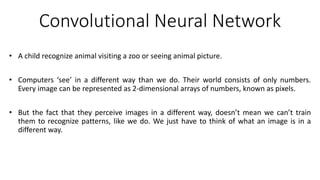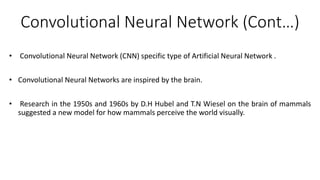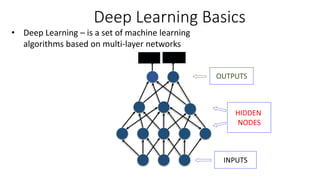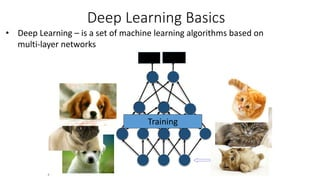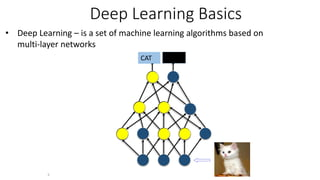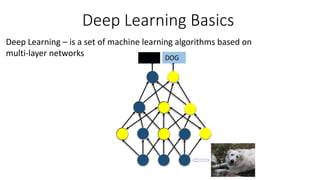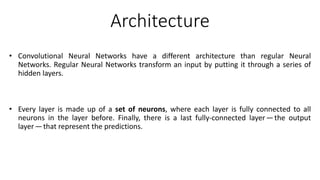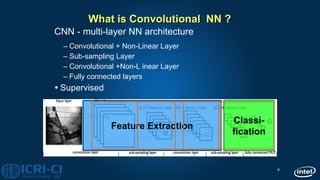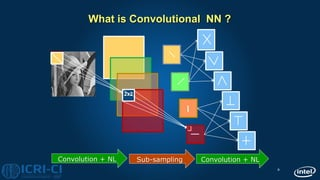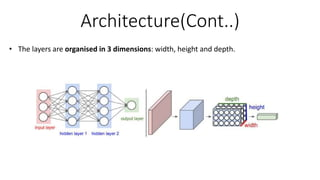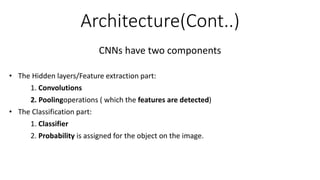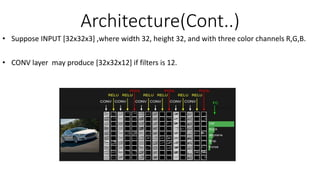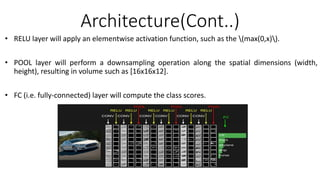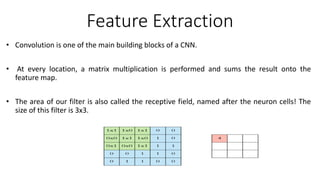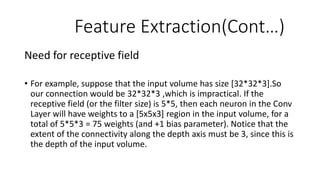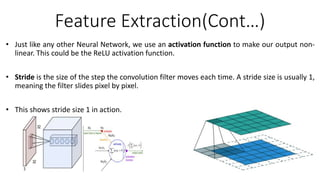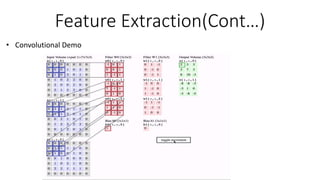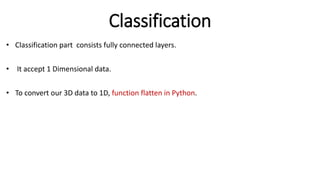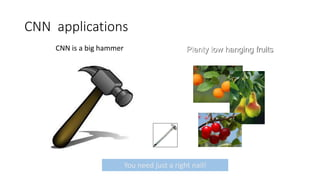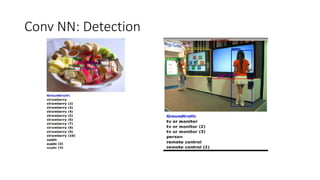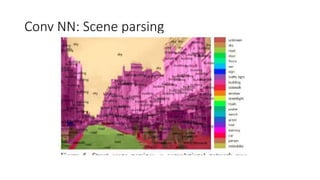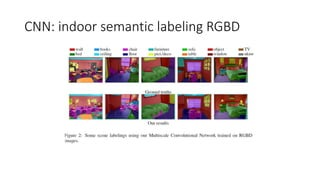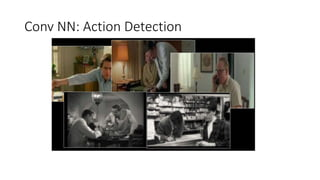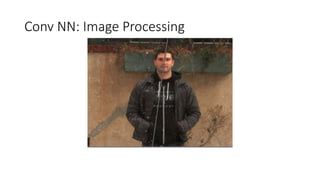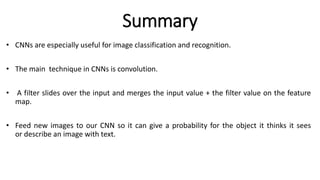1 de 26

### Convolutional neural network

• 1. Convolutional Neural Network • A child recognize animal visiting a zoo or seeing animal picture. • Computers ‘see’ in a different way than we do. Their world consists of only numbers. Every image can be represented as 2-dimensional arrays of numbers, known as pixels. • But the fact that they perceive images in a different way, doesn’t mean we can’t train them to recognize patterns, like we do. We just have to think of what an image is in a different way.
• 2. Convolutional Neural Network (Cont…) • Convolutional Neural Network (CNN) specific type of Artificial Neural Network . • Convolutional Neural Networks are inspired by the brain. • Research in the 1950s and 1960s by D.H Hubel and T.N Wiesel on the brain of mammals suggested a new model for how mammals perceive the world visually.
• 3. Deep Learning Basics OUTPUTS HIDDEN NODES CAT DOG • Deep Learning – is a set of machine learning algorithms based on multi-layer networks INPUTS
• 4. Deep Learning Basics CAT DOG • Deep Learning – is a set of machine learning algorithms based on multi-layer networks 4 Training
• 5. Deep Learning Basics CAT DOG • Deep Learning – is a set of machine learning algorithms based on multi-layer networks 5
• 6. Deep Learning Basics CAT DOG Deep Learning – is a set of machine learning algorithms based on multi-layer networks
• 7. Architecture • Convolutional Neural Networks have a different architecture than regular Neural Networks. Regular Neural Networks transform an input by putting it through a series of hidden layers. • Every layer is made up of a set of neurons, where each layer is fully connected to all neurons in the layer before. Finally, there is a last fully-connected layer —the output layer —that represent the predictions.
• 8. 8 What is Convolutional NN ? CNN - multi-layer NN architecture – Convolutional + Non-Linear Layer – Sub-sampling Layer – Convolutional +Non-L inear Layer – Fully connected layers  Supervised Feature Extraction Classi- fication
• 9. 9 What is Convolutional NN ? 2x2 Convolution + NL Sub-sampling Convolution + NL
• 10. Architecture(Cont..) • The layers are organised in 3 dimensions: width, height and depth.
• 11. Architecture(Cont..) CNNs have two components • The Hidden layers/Feature extraction part: 1. Convolutions 2. Poolingoperations ( which the features are detected) • The Classification part: 1. Classifier 2. Probability is assigned for the object on the image.
• 12. Architecture(Cont..) • Suppose INPUT [32x32x3] ,where width 32, height 32, and with three color channels R,G,B. • CONV layer may produce [32x32x12] if filters is 12.
• 13. Architecture(Cont..) • RELU layer will apply an elementwise activation function, such as the (max(0,x)). • POOL layer will perform a downsampling operation along the spatial dimensions (width, height), resulting in volume such as [16x16x12]. • FC (i.e. fully-connected) layer will compute the class scores.
• 14. Feature Extraction • Convolution is one of the main building blocks of a CNN. • At every location, a matrix multiplication is performed and sums the result onto the feature map. • The area of our filter is also called the receptive field, named after the neuron cells! The size of this filter is 3x3.
• 15. Feature Extraction(Cont…) Need for receptive field • For example, suppose that the input volume has size [32*32*3].So our connection would be 32*32*3 ,which is impractical. If the receptive field (or the filter size) is 5*5, then each neuron in the Conv Layer will have weights to a [5x5x3] region in the input volume, for a total of 5*5*3 = 75 weights (and +1 bias parameter). Notice that the extent of the connectivity along the depth axis must be 3, since this is the depth of the input volume.
• 16. Feature Extraction(Cont…) • Just like any other Neural Network, we use an activation function to make our output non- linear. This could be the ReLU activation function. • Stride is the size of the step the convolution filter moves each time. A stride size is usually 1, meaning the filter slides pixel by pixel. • This shows stride size 1 in action.
• 17. Feature Extraction(Cont…) • Because the size of the feature map is always smaller than the input, we have to do something to prevent our feature map from shrinking. This is where we use padding. • Pooling layer reduce the dimensionality of parameters and computation in the network. • The most frequent type of pooling is max pooling, which takes the maximum value in each window.
• 18. Feature Extraction(Cont…) • Convolutional Demo
• 19. Classification • Classification part consists fully connected layers. • It accept 1 Dimensional data. • To convert our 3D data to 1D, function flatten in Python.
• 20. CNN applications CNN is a big hammer Plenty low hanging fruits You need just a right nail!
• 21. Conv NN: Detection
• 22. Conv NN: Scene parsing
• 23. CNN: indoor semantic labeling RGBD
• 24. Conv NN: Action Detection
• 25. Conv NN: Image Processing
• 26. Summary • CNNs are especially useful for image classification and recognition. • The main technique in CNNs is convolution. • A filter slides over the input and merges the input value + the filter value on the feature map. • Feed new images to our CNN so it can give a probability for the object it thinks it sees or describe an image with text.